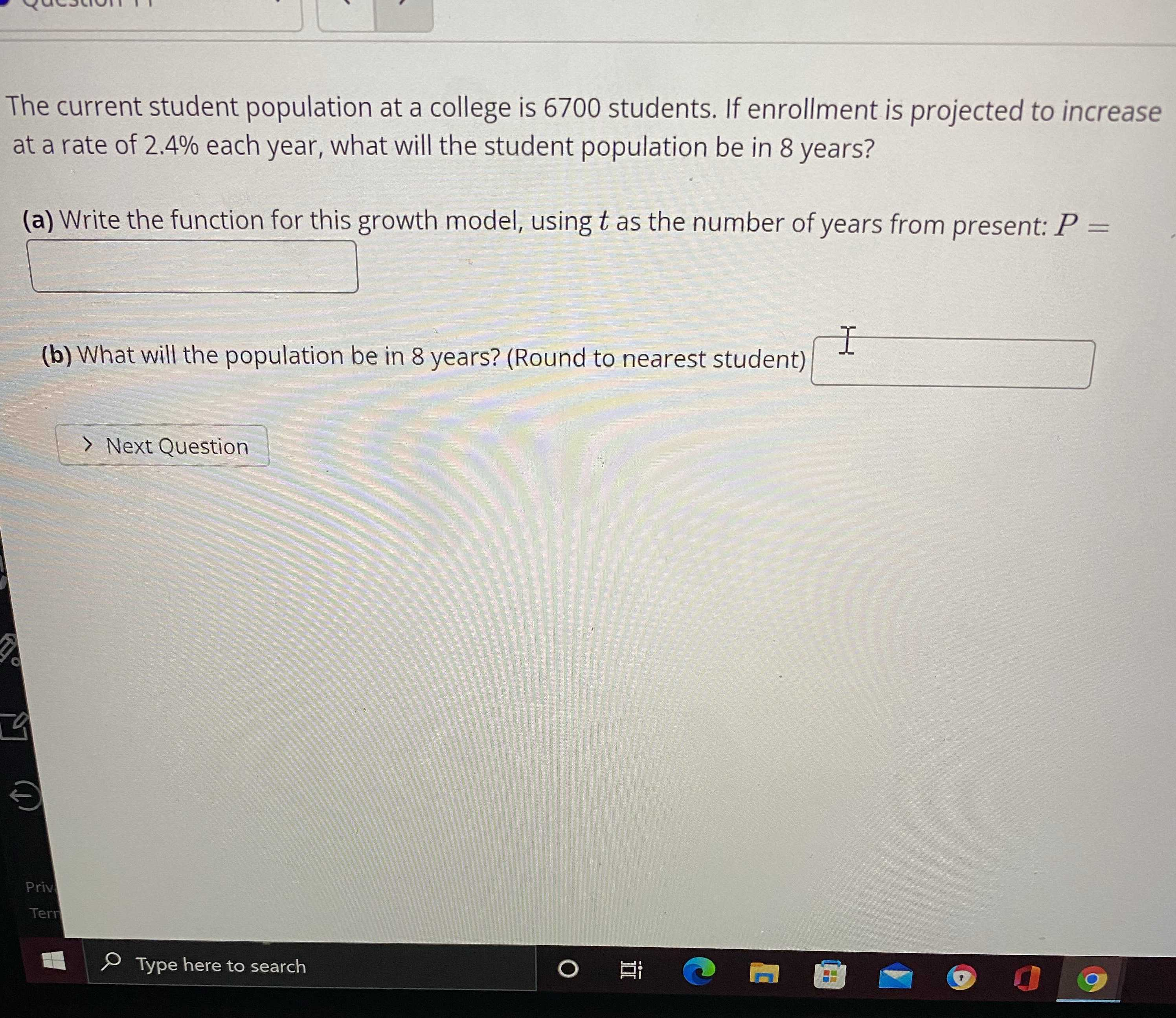### Still have math questions?

Algebra
QuestionThe current student population at a college is $$6700$$ students. If enrollment is projected to increase at a rate of $$2.4 \%$$ each year, what will the student population be in $$8$$ years?

(a) Write the function for this growth model, using $$t$$ as the number of years from present:

$$P =$$ _____

(b) What will the population be in $$8$$ years? (Round to nearest student)

6700*（1+2.4/100）$$^{t}$$
$$\approx 8100$$